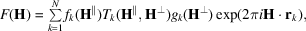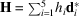International
Tables for
Crystallography
Volume B
Reciprocal space
Edited by U. Shmueli

International Tables for Crystallography (2010). Vol. B, ch. 4.6, pp. 611-612   | 1 | 2 |

## Section 4.6.3.3.2.3. Structure factor

W. Steurera* and T. Haibacha

aLaboratory of Crystallography, Department of Materials, ETH Hönggerberg, HCI G 511, Wolfgang-Pauli-Strasse 10, CH-8093 Zurich, Switzerland
Correspondence e-mail:  walter.steurer@mat.ethz.ch

#### 4.6.3.3.2.3. Structure factor

| top | pdf |

The structure factor for the decagonal phase corresponds to the Fourier transform of the 5D unit cell,with 5D diffraction vectors, N hyperatoms, parallel-space atomic scattering factor, temperature factorand perpendicular-space geometric form factor.is equivalent to the conventional Debye–Waller factor anddescribes random fluctuations along the perpendicular-space coordinate. These fluctuations cause characteristic jumps of vertices in physical space (phason flips). Even random phason flips map the vertices onto positions which can still be described by physical-space vectors of the type. Consequently, the setof all possible vectors forms amodule. The shape of the atomic surfaces corresponds to a selection rule for the positions actually occupied. The geometric form factoris equivalent to the Fourier transform of the atomic surface, i.e. the 2D perpendicular-space component of the 5D hyperatoms.

For example, the canonical Penrose tilingcorresponds to the Fourier transform of pentagonal atomic surfaces:whereis the area of the 5D unit cell projected uponandis the area of the kth atomic surface. The areacan be calculated using the formulaEvaluating the integral by decomposing the pentagons into triangles, one obtainswithrunning over the five triangles, where the radii of the pentagons are,,and the vectorsAs shown by Ishihara & Yamamoto (1988), the Penrose tiling can be considered to be a superstructure of a pentagonal tiling with only one type of pentagonal atomic surface in the nD unit cell. Thus, for the Penrose tiling, three special reflection classes can be distinguished: forandthe class of strong main reflections is obtained, and forthe classes of weaker first- and second-order satellite reflections are obtained (see Fig. 4.6.3.18).

### References

Ishihara, K. N. & Yamamoto, A. (1988). Penrose patterns and related structures. I. Superstructure and generalized Penrose patterns. Acta Cryst. A44, 508–516.